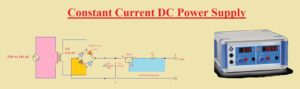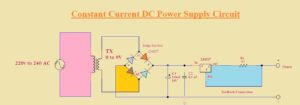Hello, readers welcome to new post. Today we will discuss Constant Current DC Power Supply Circuit. The constant current power supply is considered as 4 pins active device. Its input is denoted as dc volts source for basic current regulators and it denotes the AC line voltage for such supply that comes with rectifiers and filters. In the case of practical operation transformer, rectifiers and filters is used for every device to generate dc supply. In the end, the power controller is connected.

In a circuit of constant power supply, there is the dc power source that operates the series pass transformer that is regulated through the high-gain operational amplifier. The feedback signals get through the use of shunt resistance Rs. In this post, we will make a circuit of a constant dc power supply through the use of an LM317 regulator.

### Introduction to LM317

• The LM317 is a variable positive voltage regulator that was created by Bob Dobkin in 1976.
• The negative correspondent of LM317 is LM337 which varies volts less than a reference.
• It comes with three pins structure used for voltage regulation.
• Through the use of this component, we can get up to 37 volts regulation according to circuit design.
• In this component circuit, there is no need for external resistance. Through this module line regulation value is about 0.01 percent and load regulation is about 0.1 percent.
• Its common specification are protection from overload current

###Features of LM317

• The main features of this module are described here
It has a value of output current 1.5 amperes
• Used to protect the overload thermal
• It helps to sustain the inner short circuit current limiting area
• It is used for floating operating in case of larger voltage circuits.
• It comes with D2PAK-3 packaging

### Constant Current DC Power Supply Circuit Specification

• Features of this circuit are discussed here
• Bridge circuit used for rectification
• A transformer of a step-down type is used
• Input volts for this circuit are 220 to 240
• 125 mA current is getting at the output load
• It gives the constant power at the load end

### Component of Project

• The main component of this circuit are listed here
• Resistances
• Capacitor
• LM317
• Transformer

### Circuit Explanation• To make a constant current dc power supply first of all make the rectifier circuit through the use of four diodes. Here we will use a bridge rectifier that will convert full ac into dc. This rectifier is linked to the output of the transformer.
• In this circuit at input, 230V input is linked that will be converted into nine volts at the secondary side of the transformer that volts are given to rectifier connected to the transformer that will convert that volt to DC volts after that these volt passes through capacitors that will remove the ripples to make the pure DC. This DC is given to voltage regulator LM317
• The LM317 is attached to the load through resistance denoted as Rx and operating as feedback of current
• Here is the formula used for the calculation of the value of resistance RX on the basis of current for output.
• In this circuit, we used 10ohm/5watt resistance and we get 125mA constant current output at our dc supply
• Iout=Vref/Rx
• If we put values of our components that are
• Iout=1.25V/10
• here Vref for LM317 is 1.25 volts
• So our final result is
• Iout = 125 mA